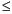Functions and CALL Routines

# RANPOI Function

Returns a random variate from a Poisson distribution.
 Category: Random Number Tip: If you want to change the seed value during execution, you must use the CALL RANPOI routine instead of the RANPOI function.

## Syntax

 RANPOI(seed,m)

### Arguments

seed

is a numeric constant, variable, or expression with an integer value. If seed0, the time of day is used to initialize the seed stream.

m

is a numeric constant, variable, or expression that specifies the mean of the distribution.

 Range: m0

The RANPOI function returns a variate that is generated from a Poisson distribution with mean m. For m < 85, an inverse transform method applied to a RANUNI uniform variate is used (Fishman 1976) (See References). For m85, the normal approximation of a Poisson random variable is used. To expedite execution, internal variables are calculated only on initial calls (that is, with each new m).

For a discussion about seeds and streams of data, as well as examples of using the random-number functions, see Generating Multiple Variables from One Seed in Random-Number Functions.

The CALL RANPOI routine, an alternative to the RANPOI function, gives greater control of the seed and random number streams.

Functions and CALL routines:Previous Page | Next Page | Top of Page